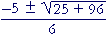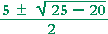# Lesson 37, Quadratic equations:  Section 2

Back to Section 1

Completing the square

The discriminant

IN LESSON 18 we saw a technique called completing the square. We will now apply it to solving a quadratic equation.

Completing the square

If we try to solve this quadratic equation by factoring,

 x2 + 6x + 2 = 0 we cannot.  Therefore, we will complete the square.  We will make the quadratic into the form a2 + 2ab + b2 = (a + b)2 .

This technique is valid only when the coefficient of x2 is 1.

1)   Transpose the constant term to the right

x2 + 6x = −2.

 2) Add a square number to both sides -- add the square of half the coefficient of x.  In this case, add the square of half of 6; that is, add the square of 3, which is 9:

x2 + 6x + 9 = −2 + 9.

The left-hand side is now the perfect square of  (x + 3).

(x + 3)2  =  7.

3 is half of the coefficient 6.

That equation has the form

 a2 = b which implies a = ±. Therefore, x + 3 = ±x = −3 ±.

That is, the solutions to

x2 + 6x + 2  =  0

are the conjugate pair,

−3 +,  −3 −.

For a method of checking these roots, see the theorem of the sum and product of the roots:  Lesson 10 of Topics in Precalculus,

In Lesson 18 there are examples and problems in which the coefficient of x is odd.  Also, some of the quadratics below have complex roots, and some involve simplifying radicals.

Problem 6.   Solve each quadratic equation by completing the square.

over the colored area.
Do the problem yourself first!

 a) x2 − 2x − 2 = 0 b) x2 − 10x + 20 = 0 x2 − 2x = 2 x2 − 10x = −20 x2 − 2x + 1 = 2 + 1 x2 − 10x + 25 = −20 + 25 (x − 1)2 = 3 (x − 5)2 = 5 x − 1 = ±x − 5 = ±x = 1 ±x = 5 ±c) x2 − 4x + 13 = 0 d) x2 + 6x + 29 = 0 x2 − 4x = −13 x2 + 6x = −29 x2 − 4x + 4 = −13 + 4 x2 + 6x + 9 = −29+ 9 (x − 2)2 = −9 (x + 3)2 = −20 x − 2 = ±3i x + 3 = ±x = 2 ± 3i x = −3 ± 2ie) x2 − 5x − 5 = 0 f) x2 + 3x + 1 = 0 x2 − 5x = 5 x2 + 3x = −1 x2 − 5x + 25/4 = 5 + 25/4 x2 + 3x + 9/4 = −1 + 9/4 (x − 5/2)2 = 5 + 25/4 (x + 3/2)2 = − 1 + 9/4 x − 5/2 = ±x + 3/2 = ±x =x =Problem 7.   Find two numbers whose sum is 10 and whose product is 20.

x = 5 ±According to the theorem of the sum and products of the roots, they are the solutions to Problem 6b above.

Here is a formula for finding the roots of any quadratic. It is proved by completing the squareIn other words, the quadratic formula completes the square for us.

Theorem.    If

ax2 + bx + c = 0,

thenThe two roots are on the right. One root has the plus sign; the other, the minus sign.  If the square root term is irrational, then the two roots are a conjugate pair.

If we call those two roots r1 and r2 , then the quadratic can be factored as

(xr1)(xr2).

We will prove the quadratic formula below.

3x2 + 5x − 8  =  0

Solution.   We have:  a = 3,  b = 5,  c = −8.

Therefore, according to the formula:

 x ====That is,

 x = −5 + 11     6 or −5 − 11     6
 x = 66 or −16  6
 x = 1 or   − 83 .

Those are the two roots.  And they are rational.  When the roots are rational, we could have solved the equation by factoring, which is always the simplest method.

 3x2 + 5x − 8 = (3x + 8)(x − 1) x = − 83 or  1.

Problem 8.   Use the quadratic formula to find the roots of each quadratic.

a)   x2 − 5x + 5

a  =  1,  b  = −5,  c  =  5.

 x ===b)  2x2 − 8x + 5

a  =  2,  b  = −8,  c  =  5.

 x ===== 2 ± ½c)  5x2 − 2x + 2

a  =  5,  b  = −2,  c  =  2.

 x ==== 2 ± 6i   10 = 1 ± 3i   5

The discriminant

The radicand  b2 − 4ac  is called the discriminant.  If the discriminant is

 A square number: The roots are rational. Positive but not a square number: The roots are real and conjugate. Negative: The roots are complex and conjugate. Zero: The roots are rational and equal -- i.e. a double root.

Problem 9.   Show:  If the roots of ax2 + bx + c are complex, and a, b, c are positive, then

2ab + c > 0.

Since the roots are complex, then the discriminant b2 − 4ac < 0. That implies  b2 < 4ac.

Now, 2ab + c > 0  if and only if

b < 2a + c

if and only if

b2 < 4a2 + 4ac + c2,

which is true.  For, since b2 < 4ac, it is less than 4ac plus a positive quantity.  4a2 and c2 are positive.

The student should be familiar with the logical expression

To prove the quadratic formula, we complete the square.  But to do that, the coefficient of x2 must be 1.  Therefore, we will divide both sides of the original equation by a:on multiplying both c and a by 4a, thus making the denominators the same (Lesson 23),Section 3:  The graph of  y = A quadratic

Back to Section 1Please make a donation to keep TheMathPage online.
Even \$1 will help.